PrinciplesRandomFields - MRC CBU Imaging Wiki
location: PrinciplesRandomFields

# Thresholding with Random Field Theory

A few notes to begin. First: you can easily read this page without knowing any matlab programming, but you may gain extra benefit if you read it with the matlab code that will generate the figures. This code is contained in the matlab scripthttp://imaging.mrc-cbu.cam.ac.uk/scripts/randomtalk.m. Second: some of the figures do not display well. Please click on any figure to get a pdf file that has much better detail. Last, this page is based primarily on the Worsley 1992 paper (see refs below). For me, this paper is the most comprehensible of the various treatments of this subject. Please refer to this paper and the Worsley 1996 paper for more detail on the issues here discussed.

See also An introduction to multiple hypothesis testing and brain data by Federico Turkeimer for a general introduction to the multiple comparison.

This page became the Random fields introduction chapter in the the Human Brain Function second edition - please see that chapter for a more up to date and better presented version of this page (but without the script!).

## Introduction

Most statistics packages for functional imaging data create statistical parametric maps. These maps have a value for a certain statistic at each voxel in the brain, which is the result of the statistical test done on the scan data for that voxel, across scans. For SPM96, this statistic is a Z statistic (see my SPM statistics tutorial ).

The null hypothesis for a particular statistical comparison probably will be that there is no change anywhere in the brain. For example, in a comparison of activation against rest, the null hypothesis would be that there are no differences between the scans in the activation condition, and the scans in the rest condition. This null hypothesis implies that the volume of Z scores for the comparison will be similar to a equivalent set of numbers from a random normal distribution.

## The multiple comparison problem

The question then becomes; how do we decide whether some of the Z statistics we see in our SPM96 map are larger (more positive) than we would expect in a similar volume of random numbers?So, in a typical SPM Z map, we have, say, 200000 Z scores. Because we have so many Z scores, even if the null hypothesis is true, we can be sure that some of these Z scores will appear to be significant at standard statistical thresholds for the the individual Z scores, such as p<0.05 or p<0.01. These p values are eqivalent to Z = 1.64 and 2.33 respectively - see the http://imaging.mrc-cbu.cam.ac.uk/scripts/randomtalk.m file.

So, if we tell SPM to show us only Z scores above 2.33, we would expect a number of false positives, even if the null hypothesis is true. So, how high should we set our Z threshold, so that we can be confident that the remaining peak Z scores are indeed too high to be expected by chance? This is the multiple comparison problem.

## Why not a Bonferroni correction?

The problem of false positives with multiple statistical tests is an old one. One standard method for dealing with this problem is to use the Bonferroni correction. For the Bonferroni correction, you set your p value threshold for accepting a test as being significant as alpha / (number of tests), where alpha is the false positive rate you are prepared to accept. Alpha is often 0.05, or one false positive in 20 repeats of your experiment. Thus, for an SPM with 200000 voxels, the Bonferroni corrected p value would be 0.05 / 200000 = [equivalent Z] 5.03. We could then threshold our Z map to show us only Z scores higher than 5.03, and be confident that all the remaining Z scores are unlikely to have occurred by chance. For some functional imaging data this is a perfectly reasonable approach, but in most cases the Bonferroni threshold will be considerably too conservative. This is because, for most SPMs, the Z scores at each voxel are highly correlated with their neighbours.

## Spatial correlation

Functional imaging data usually have some spatial correlation. By this, we mean that data in one voxel are correlated with the data from the neighbouring voxels. This correlation is caused by several factors: * With low resolution imaging (such as PET and lower resolution fMRI) data from an individual voxel will contain some signal from the tissue around that voxel;

• The reslicing of the images during preprocessing causes some smoothing across voxels;
• Most SPM analyses work on smoothed images, and this creates strong spatial correlation (see my smoothing tutorial for further explanation). Smoothing is often used to improve signal to noise.

The reason this spatial correlation is a problem for the Bonferroni correction is that the Bonferroni correction assumes that you have performed some number of independent tests. If the voxels are spatially correlated, then the Z scores at each voxel are not independent. This will make the correction too conservative.

## Spatial correlation and independent observations

An example can show why the Bonferroni correction is too conservative with non-independent tests. The code for the following figures is in thehttp://imaging.mrc-cbu.cam.ac.uk/scripts/randomtalk.mfile. Let us first make an example image out of random numbers. We generate 16384 random numbers, and then put them into a 128 by 128 array. This results in a 2D image of spatially independent random numbers. Here is an example: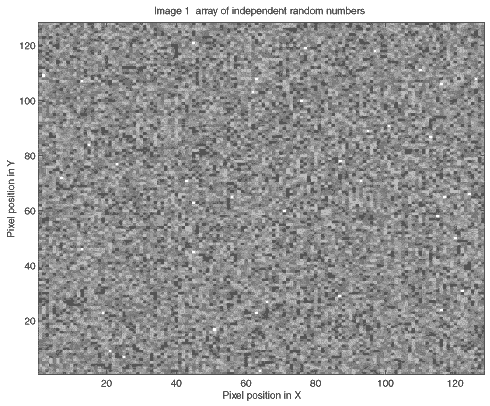In this picture, whiter pixels are more positive, darker pixels more negative. The Bonferroni correction is appropriate for this image, because the image is made up of 128*128 = 16384 random numbers from a normal distribution. Therefore, from the Bonferroni correction (alpha / N = 0.05 / 16384 = [Z equivalent] 4.52), we would expect only 5 out of 100 such images to have one or more random numbers in the whole image larger than 4.52.

The situation changes if we add some spatial correlation to this image. We can take our image above, and perform the following procedure:

• Break up the image into 8 by 8 squares;
• For each square, calculate the mean of all 64 random numbers in the square;
• Replace the 64 random numbers in the square by the mean value.

(In fact, we have one more thing to do to our new image values. When we take the mean of 64 random numbers, this mean will tend to zero. We have therefore to multiply our mean numbers by 8 to restore a variance of 1. This will make the numbers correspond to the normal distribution again).This is the image that results from following the above procedure on our first set of random numbers: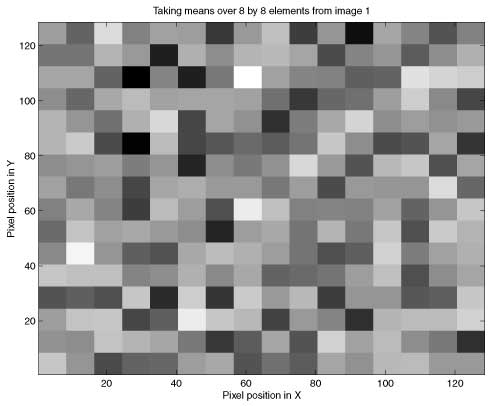We still have 16384 numbers in our image. However, it is clear that we now have only (128 / 8) * (128 / 8) = 256 independent numbers. The appropriate Bonferroni correction would then be (alpha / N = 0.05 / 256 = [Z equivalent] 3.55). We would expect that if we took 100 such mean-by-square-processed random number images, then only 5 of the 100 would have a square of values greater than 3.55 by chance. However, if we took the original Bonferroni correction for the number of pixels rather than the number of independent pixels, then our Z threshold would be far too conservative.

## Smoothed images and independent observations

The mean-by-square process we have used above is a form of smoothing (see the smoothing tutorial for details). In the mean-by-square case, the averaging takes place only within the squares, but in the case of smoothing with a kernel, the averaging takes place in a continuous way across the image. Here is our first random number image smoothed with a Gaussian kernel of FWHM 8 by 8 pixels: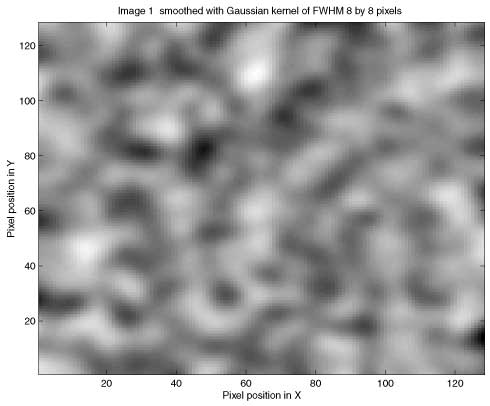(As for the mean-by-square example, the smoothing reduces the variance of the numbers in the image, because an average of random numbers tends to zero. In order to return the variance of the numbers in the image to one, to match the normal distribution, the image must be multiplied by a scale factor. The derivation of this scaling factor is rather technical, and not relevant to our discussion here. You will find the code in http://imaging.mrc-cbu.cam.ac.uk/scripts/randomtalk.m).

In our smoothed image, as for the mean-by-square image, we no longer have 16384 independent observations, but some smaller number, because of the averaging across pixels. If we knew how many independent observations there were, we could use a Bonferroni correction as we did for the mean-by-square example. Unfortunately it is not easy to work out how many independent observations there are in a smoothed image. So, we must take a different approach to determine our Z score threshold. The approach used by SPM and other packages is to use Random Field Theory (RFT).

## Using Random Field Theory

You can think of the application of RFT as proceeding in three steps. First, you determine how manyreselsthere are in your image. Then you use the resel count and some sophisticated maths to work out the expectedEuler characteristic(EC) of your image, when it is thresholded at various levels. These expected ECs can be used to give the correct threshold for the required control of false positives (alpha).

## What is a resel?

A resel is a "resolution element". The number of resels in an image is similar to the number of independent observations in the image. However, they are not the same, as we will see below. A resel is defined as a block of pixels of the same size as the FWHM of the smoothness of the image. In our smoothed image above, the smoothness of the image is 8 pixels by 8 pixels (the smoothing that we applied). A resel is therefore a 8 by 8 pixel block, and the number of resels in our image is (128 / 8) * (128 / 8) = 256. Note that the number of resels depends only on the number of pixels, and the FWHM.

## What is the Euler characteristic?

The Euler characteristic of an image is a property of the image after it has been thresholded. For our purposes, the EC can be thought of as the number of blobs in an image after it has been thresholded. This is best explained by example. Let us take our smoothed image, and threshold it at Z greater than 2.75. This means we set to zero all the pixels with Z scores less than or equal to 2.75, and set to one all the pixels with Z scores greater than 2.75. If we do this to our smoothed image, we get the image below. Zero in the image displays as black and one as white.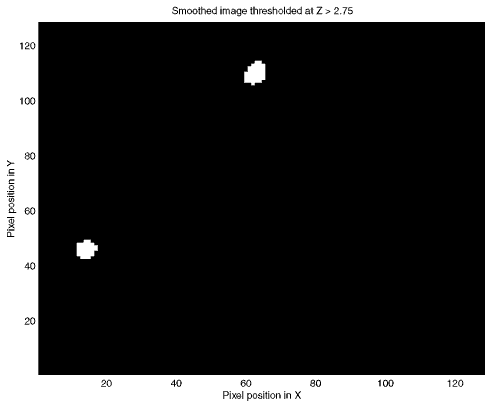In this picture, there are two blobs, corresponding to two areas with Z scores higher than 2.75. The EC of this image is therefore 2. If we increase the threshold to 3.5, we find that the lower left hand blob disappears (the highest Z in the peak was less than 3.5).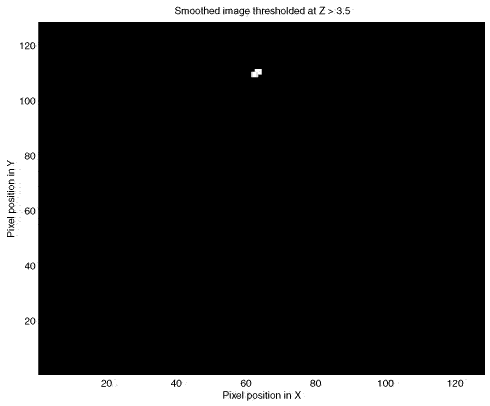The upper central blob remains; the EC of the image above is therefore 1. It turns out that if we know the number of resels in our image, it is possible to estimate the most likely value of the EC at any given threshold. The formula for this estimate, for two dimensions, is on page 906 of Worsley 1992, and is implemented in http://imaging.mrc-cbu.cam.ac.uk/scripts/randomtalk.m to create the graph below. The graph shows the expected EC of our smoothed image, of 256 resels, when thresholded at different Z values.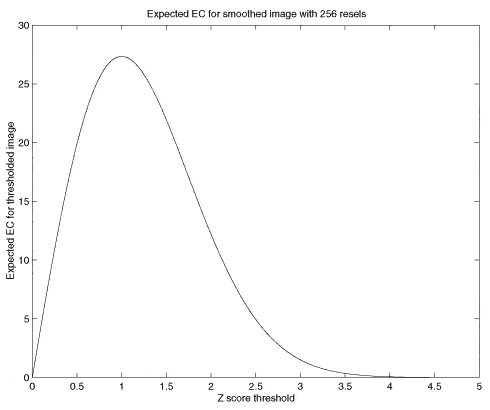Note that the graph does a reasonable job of predicting the EC in our image; at Z = 2.75 threshold it predicted an EC of 2.8, and at a Z of 3.5 it predicted an EC of 0.3.

## How does the Euler characteristic give a Z threshold?

The useful feature of the expected EC is this: when the Z thresholds become high and the predicted EC drops towards zero, the expected EC is a good approximation of the probability of observing one or more blobs at that threshold. So, in the graph above, when the Z threshold is set to 4, the expected EC is 0.06. This can be rephrased thus: the probability of getting one or more regions where Z is greater than 4, in a 2D image with 256 resels, is 0.06. So, we can use this for thresholding. If x is the Z score threshold that gives an expected EC of 0.05, then, if we threshold our image at x, we can expect that any blobs that remain have a probability of less than or equal to 0.05 that they have occurred by chance.Note that this threshold, x, depends only on the number of resels in our image.

## How does the Random Field maths compare to the Bonferroni correction?

I stated above that the resel count in an image is not exactly the same as the number of independent observations. If it was the same, then instead of using RFT for the expected EC, we could use a Bonferroni correction for the number of resels. However, these two corrections give different answers. Thus, for an alpha of 0.05, the Z threshold according to RFT, for our 256 resel image, is Z=4.06. However, the Bonferroni threshold, for 256 independent tests, is 0.05/256 = [Z equivalent] 3.55. So, although the RFT maths gives us a Bonferroni-like correction, it is not the same as a Bonferroni correction. It is easy to show that the RFT correction is better than a Bonferroni correction, by simulation. Using the code in thehttp://imaging.mrc-cbu.cam.ac.uk/scripts/randomtalk.m, you can repeat the creation of smoothed random images many times, and show that the RFT threshold of 4.06 does indeed give you about 5 images in 100 with a significant Z score peak.

## To three dimensions

Exactly the same principles apply to a smoothed random number image in three dimensions. In this case, the EC is the number of 3D blobs - perhaps "globules" - of Z scores above a certain threshold. Pixels might better be described as voxels (pixels with volume). The resels are now in 3D, and one resel is a cube of voxels that is of size (FWHM in x) by (FWHM in y) by (FWHM in z). The formula for the expected EC is different in the 3D case, but still depends only on the resels in the image. Now, if we find the threshold giving an expected EC of 0.05, in 3D, we have a threshold above which we can expect that any remaining Z scores are unlikely to have occurred by chance, with a p<0.05.

## Random fields and SPM96

It is exactly this technique that is used to give corrected p values in SPM96. There is only one slight variation from the discussion above, and that is that SPM96 does not assume that the brain volume is the same smoothness (FWHM) as the kernel you have used to smooth the images. Instead SPM looks at the data in the images (in fact the residuals from the statistical analysis) to calculate the smoothness. From these calculations it derives estimates for the FWHM in x, y and z.Other than this, the corrected statistics are calculated just as described above. Below is a page from an SPM96 results printout (you can click on the picture to get the page in high detail pdf format):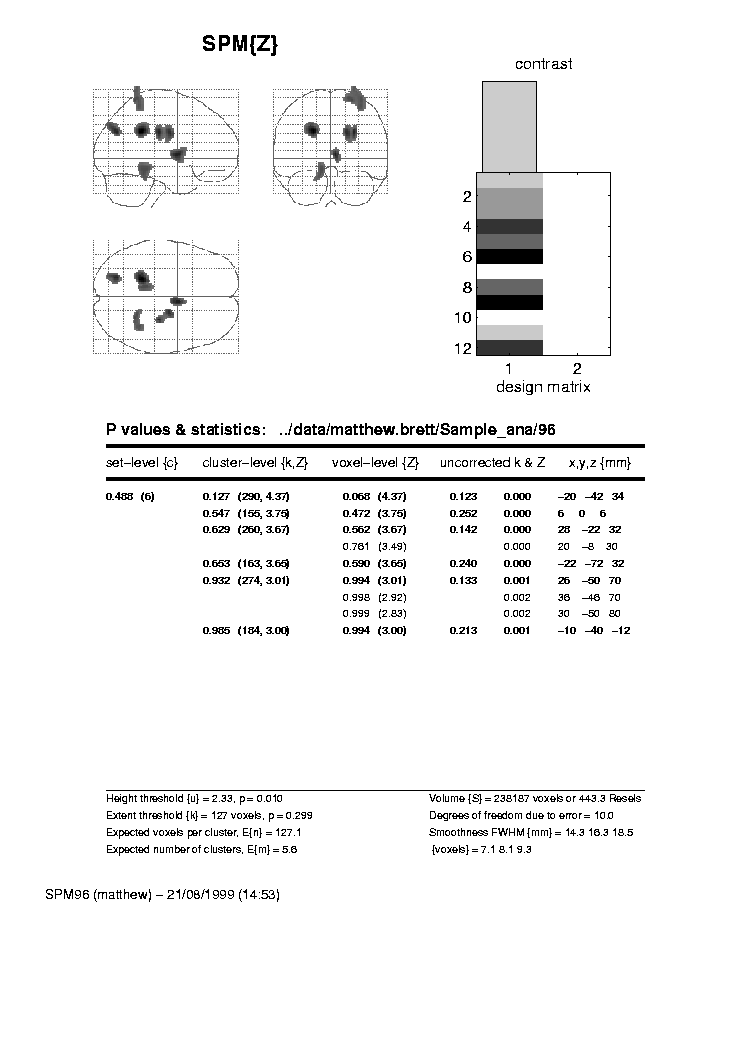You will see that the FWHM values are printed at the bottom of the page - here they are 7.1 voxels in x, 8.1 voxels in y, and 9.3 voxels in z. A resel is therefore a block of volume 7.1*8.1*9.3 = 537.3 voxels (if we use the exact FWHM values, before rounding). As there were 238187 intracerebral voxels in this analysis, this gives 238187 / 537.3 = 443.3 intracerebral resels (see the bottom of the printout). The top line of the table gives the statistics for the most significant Z score in the analysis. The middle column, labelled 'voxel-level {Z}', shows a Z score (in brackets) of 4.37. This is the Z score from the statistical analysis, before any statistical correction. The uncorrected p value, from which this Z score was derived, is shown in the column labelled (rather confusingly) 'uncorrected k & Z'. It is the right hand of the two figures in this column, just before the x, y and z coordinates of the voxel, and is 0.000. In fact, from the Z score, we can infer that the p value would have been 0.000006. The figure that we are interested in is the corrected p value for the height of the Z score, and this is the left hand value in the middle column ('voxel-level {Z}'). This figure is 0.068. 0.068 is the expected EC, in a 3D image of 443 resels, thresholded at Z = 4.37. This is equivalent to saying that the probability of getting one or more blobs of Z score 4.37 or greater, is 0.068.

There is another corrected p value that is also based on RFT, in the 'cluster level {k,Z}' column. This is the corrected p value for the number of voxels above the overall Z threshold (the 'Height threshold' at the bottom of the page - here Z = 2.33). This RFT correction is rather more complex, and I don't propose to discuss it further (you may be glad to hear). See the Friston et al paper for more details.

## More sophisticated Random Fielding

Two of the statements above are deliberate oversimplifications for the sake of clarity. Both are discussed in Worsley's 1996 paper.The first oversimplification is that the expected EC depends only on the number of resels in the image. In fact, this is an approximation, which works well when the volume that we are looking at has a reasonable number of resels. This is true for our two dimensional example, where the FWHM was 8 and our image was 128 by 128. However, the precise EC depends not only on the number of resels, but the shape of the volume in which the resels are contained. It is possible to derive a formula for the expected EC, based on the number of resels in the area we are thresholding, and the shape of the area (see Worsley 1996). This formula is more precise than the formula taking account of the number of resels alone. When the area to be thresholded is large, compared to the FWHM, as is the case when we are thresholding the whole brain, the two formulae give very similar results. However, when the volume for thresholding is small, the formulae give different results, and the shape of the area must be taken into account. This is the case when you require a threshold for a small volume, such as a region of interest. Please see my small volume correction page for more details and links to software to implement these corrections.

The second oversimplification was to state that the SPM Z statistics should be similar to an equivalent volume of random numbers on the null hypothesis. In fact, because of the way that the Z scores are derived, this is only true for quite high degrees of freedom (see the Worsley 1996 paper again). At low degrees of freedom, say less than 40, the SPM Z scores can generate an excess of false positives, using the RFT maths. It is therefore safer to generate thresholds for the t statistics that were the raw material for the SPM Z scores (see my SPM statistics tutorial ). For this, you can use RFT formulae for the expected EC of t fields (instead of Z fields) to give a more accurate threshold.

## Random fields and SPM99

SPM 99 takes into account both of the caveats in the preceding paragraph. Thus, in generating the expected EC (and corrected p value for height), it uses the raw t statistics. It also uses the EC formula that takes into account the shape of the thresholded volume. Here is an example of an SPM99b results printout. I have reproduced the SPM96 analysis above, by running the relevant contrast, selecting the spm96 default uncorrected p value as threshold (0.01), and the same voxel extent threshold (here 127). Then I clicked on the Volume button to get an SPM96-like summary of the peak voxels.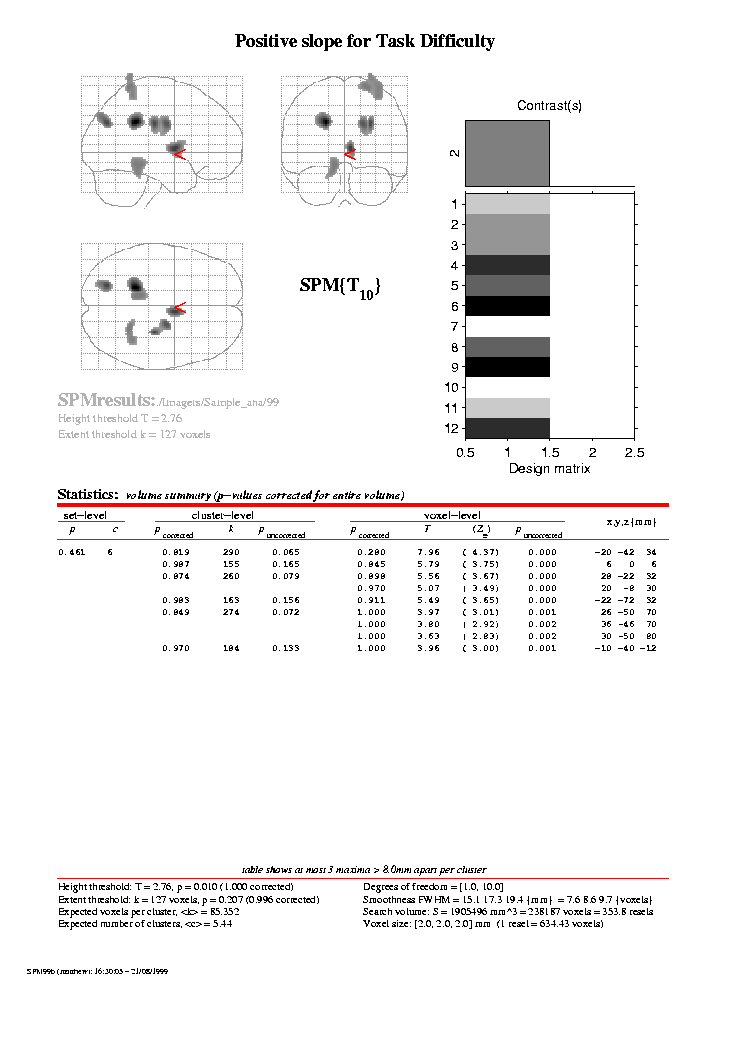The t statistic for each voxel is shown in the 'T' column, under the 'Voxel level' heading. The 'Z=' column shows the equivalent Z score, as used by SPM96. You will see these are identical to the equivalent Z scores for SPM96. There are some minor changes in the calculation of the smoothness of the data in SPM99, and this is reflected in slightly larger resel size (see the bottom of the printout). The expected ECs are shown in the 'p corrected' column, under the 'Voxel level' heading. For SPM99, the expected EC for the peak voxel is now 0.280, instead of 0.068, as it was for SPM96. This difference is almost entirely explained by the low degrees of freedom in this analysis; the degrees of freedom due to error are only 10. In SPM96, the low degrees of freedom have led to bias in the Z score generation, and the EC calculation is therefore too liberal.

## Other ways of detecting significant signal

The random fields method allows you to set a threshold allowing a known false positive rate across the whole brain. However, there are some problems with this approach. Firstly, this approach is an hypothesis testing approach rather than an estimation approach which may be more appropriate. Second, the thresholding approach does not give you a good estimate of the shape of the signal. These problems are discussed in more detail in Federico Turkheimer's tutorials: Multiple hypothesis testing and brain data

## Other sources of information

Random field theory can be rather obscure, and more difficult to follow than the creation of the statistical maps. The best introductory paper is an early paper by Keith Worsley. This paper outlines the Montreal approach to generating statistical parametric maps, which differs somewhat from that of SPM. However, the discussion of Gaussian random fields, Euler characteristics and corrected p values is very useful.

There is an overview of the field in the SPM course notes. It is very technical, and not easy reading. There are slides for the talk on this chapter, given on the 1998 SPM course. These have some good pictures illustrating the issues involved.

A more recent paper by Keith Worsley covers the maths of corrected p values in a statistical map, when you are only looking within a defined area of the map - i.e. when you have an anatomical hypothesis as to the site of your activation. See also my small volume correction page for a very brief introduction to this area, and links to software to implement the Worsley corrections.

## Conclusion

Here ends the lesson. I hope that it has been of some use. I would be very glad to hear from anyone with suggestions for improvements, detected errors, or other feedback.

MatthewBrett 19/8/99 (FB)

Worsley, K.J., Marrett, S., Neelin, P., and Evans, A.C. (1992). A three-dimensional statistical analysis for CBF activation studies in human brain. Journal of Cerebral Blood Flow and Metabolism, 12:900-918.

Worsley, K.J., Marrett, S., Neelin, P., Vandal, A.C., Friston, K.J., and Evans, A.C. (1996). A unified statistical approach for determining significant signals in images of cerebral activation. Human Brain Mapping, 4:58-73.

Friston KJ, Worsley KJ, Frackowiak RSJ, Mazziotta JC, Evans AC (1994). Assessing the Significance of Focal Activations Using their Spatial Extent. Human Brain Mapping, 1:214-220.